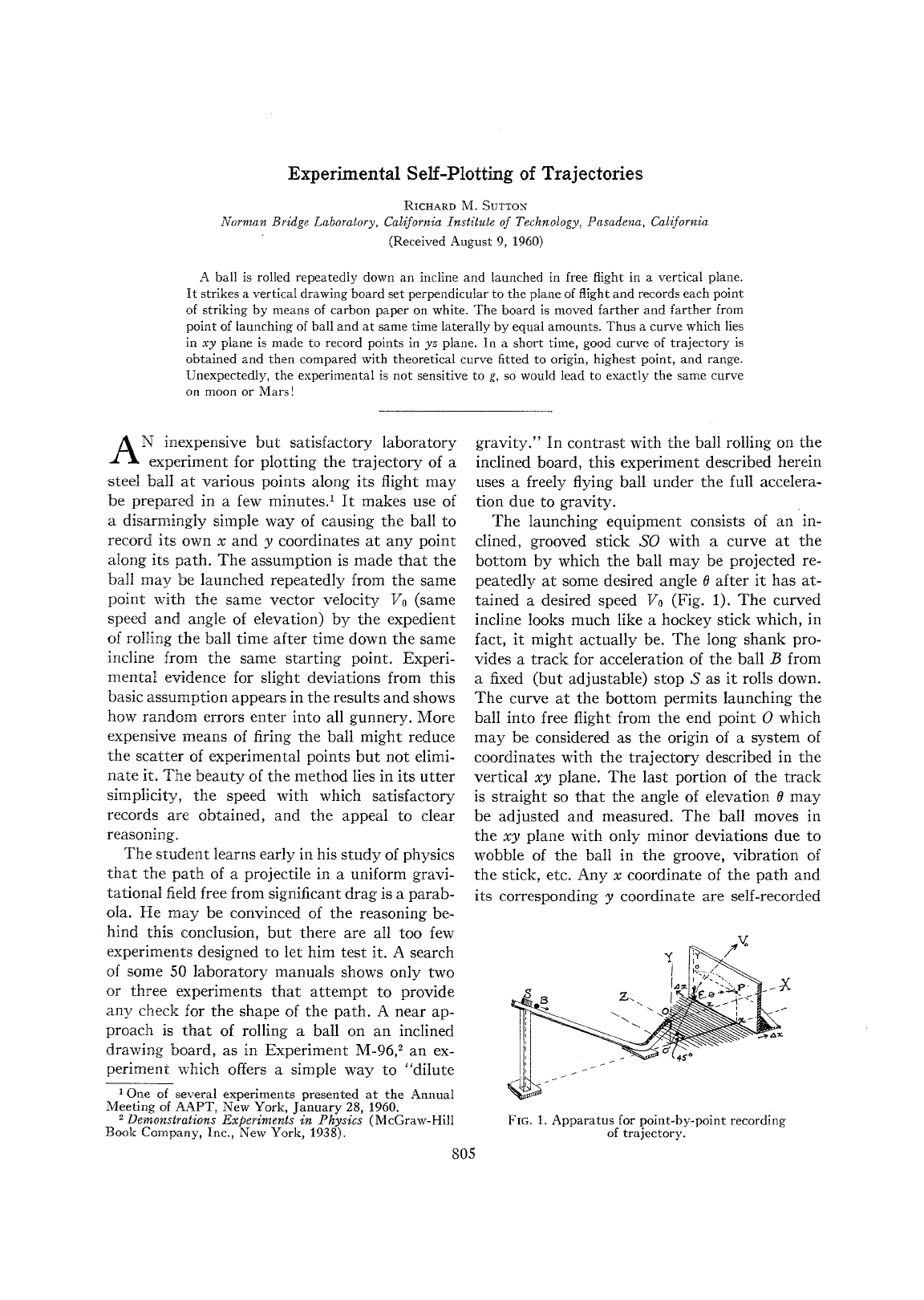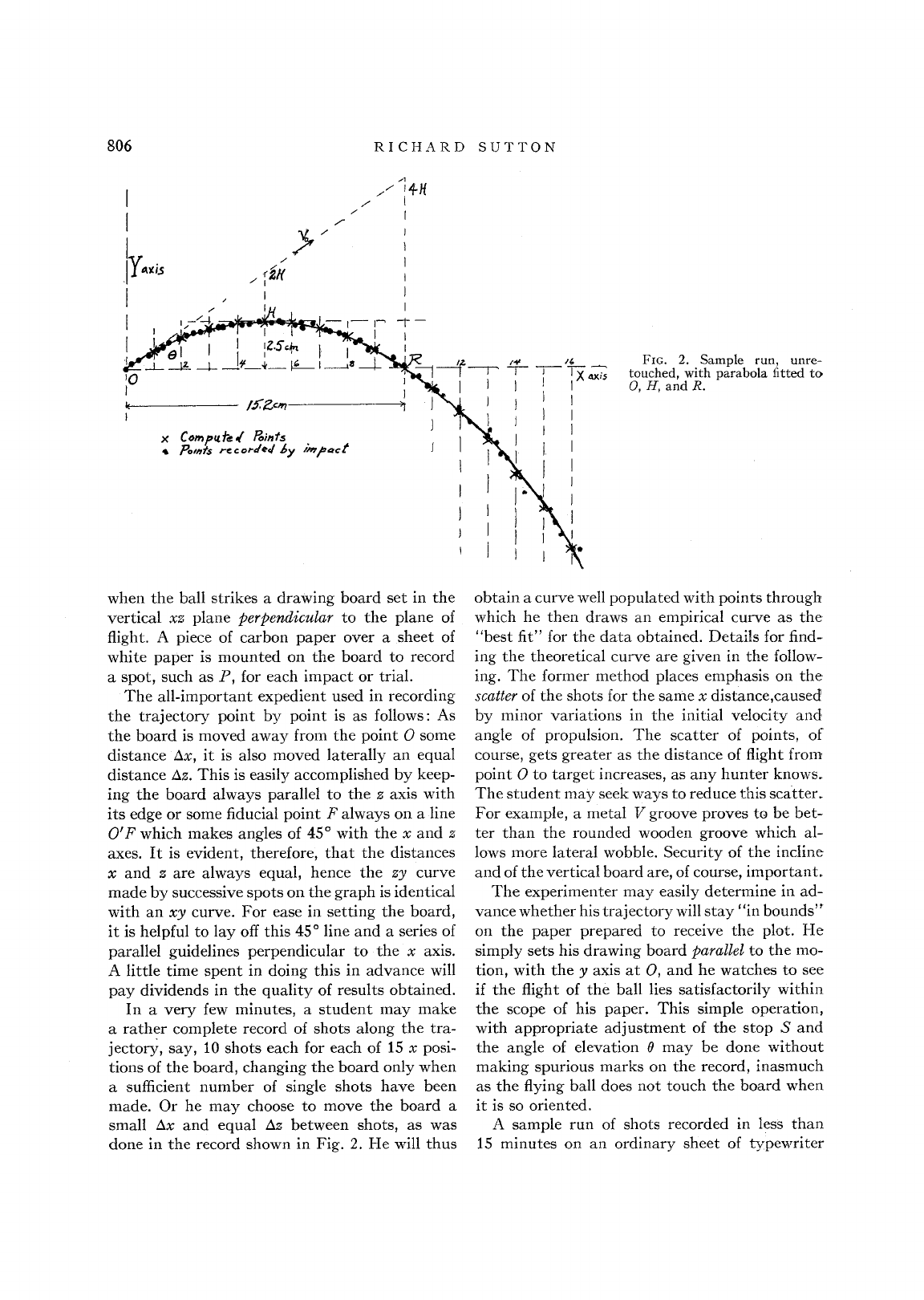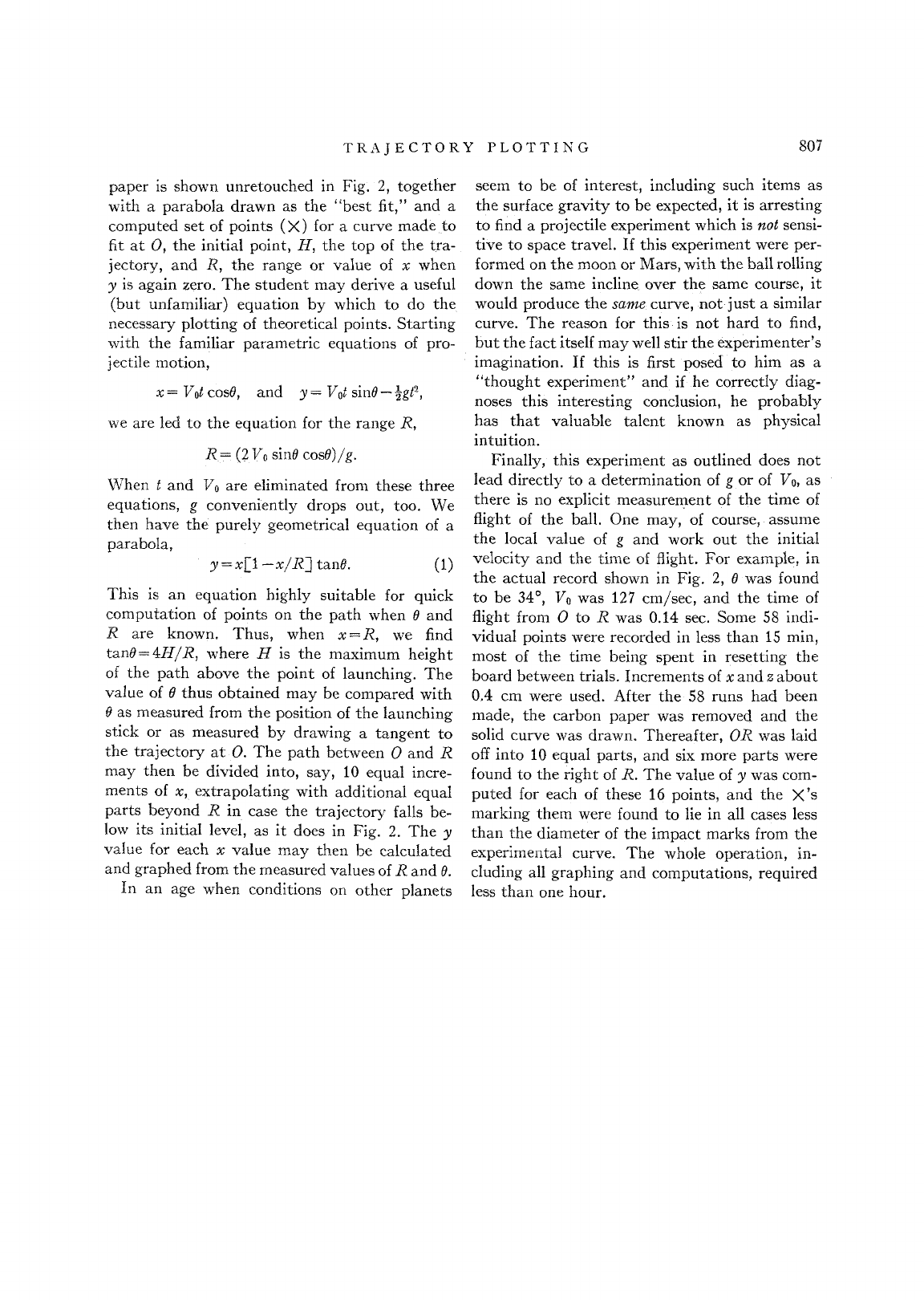### TL;DR Most people think that when studying the physics of o...
Richard Sutton (1900-1966) was a physics professor at Caltech and k...
![](https://i.imgur.com/MBvtHL6.png) To calculate the range of t...
This is a good example of a problem in which finding the solution w...
Looking at the equation for range R, the initial velocity, V_0 shou...Richard Sutton (1900-1966) was a physics professor at Caltech and known for his extraordinary imagination and ingenuity in designing lecture and laboratory experiments illustrating basic principles and phenomena in physics. His "Demonstration Experiments in Physics" has been a handbook for physics teachers since 1938. ![](https://i.imgur.com/laCOPdd.png) ![](https://i.imgur.com/MBvtHL6.png) To calculate the range of the projectile we start by determining its horizontal position $$x(t) = v_0 t \cos \alpha$$ In the vertical direction $$y(t) = v_0 t \sin \alpha - \frac{1} {2} g t^2$$ Now we need to determine the time in takes for the projectile to hit the ground ($y=0$). $$0 = v_0 t \sin \alpha - \frac{1} {2} g t^2$$ By factoring: $$t = 0$$ or $$t = \frac{2 v_0 \sin \alpha} {g}$$ The first solution corresponds to when the projectile is first launched and the second solution corresponds to the flight time before the projectile hits the ground again. If we now use this value in the horizontal equation $$R = \frac {2 v_0^2} {g} \cos \alpha \, \sin \alpha$$ By the conservation of energy $\frac{1}{2}mv_0^2 = mgh$ and so $v^2_0=2gh$, turning the range expression into $$R=4h \cos(\alpha) \sin(\alpha)$$ which has no $g$ in it! ### TL;DR Most people think that when studying the physics of objects moving in space, $g$ always shows up. In 1960, Richard Sutton wrote a paper describing a simple counterexample: if a mass slides down an inclined plane and is launched at ground level with a certain angle, the range doesn't depend on g - the outcome is the same if the experiment is performed on the Moon, Earth or on Mars. This is a good example of a problem in which finding the solution with math is substantially easier than trying to grasp it through "physical intuition". The key difference between doing this experiment on Earth vs Mars is that on the planet where g is greater, the launch speed is also greater which will compensate for the increased gravity, giving in the end the exact same range. Looking at the equation for range R, the initial velocity, V_0 should be squared in the expression given.